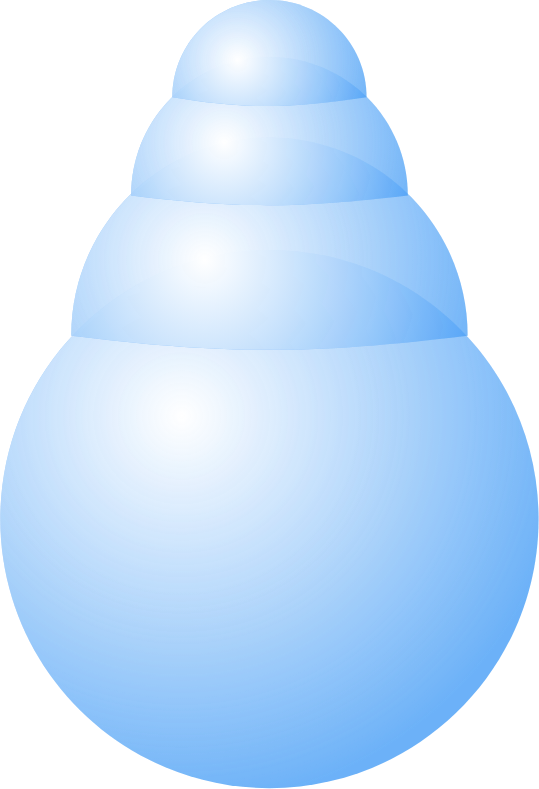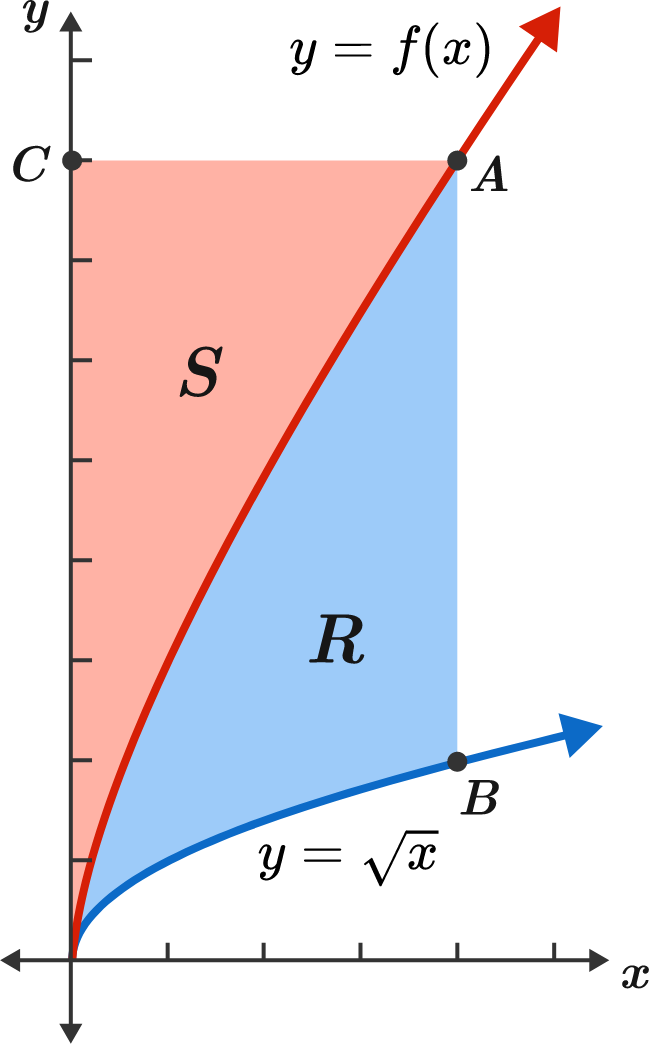# Problems of the Week

Contribute a problemA hemispherical bubble is placed on top of a spherical bubble of radius $1$.

A smaller, second hemispherical bubble is then placed on the first one. This process is continued until $n$ hemispheres are placed.

Find the maximum possible height of such a tower with $80$ hemispheres on top of the sphere.

Take a point $A$ on the graph of $y=f(x),$ and let $B$ be a point on the graph of $y=\sqrt{x}$ such that $AB$ is parallel to the $y$-axis. Call the area bounded by these two curves and the segment $AB$ as $R.$

Now, let $C$ be a point on the $y$-axis such that $AC$ is parallel to the $x$-axis. Call the area bounded by the curve $y=f(x),$ the $y$-axis, and the segment $AC$ as $S.$

Given that the function $f$ is continuous and the areas $R$ and $S$ are equal, which of the following statements is true?Let $(a_n)$ be a sequence of integers defined as $a_0 = 0; \quad a_1 = 1; \quad a_{n+1}=\text{the next integer that shares no digits with }a_n.$ How many digits does the term $a_{2018}$ have?

Hints:

1. Here are a few more terms in the sequence: $a_2=2,\ a_3=3,\ ...,\ a_9=9,\ a_{10}=10,\ a_{11}=22,\ a_{12}=30,\ a_{13}=41,\, \ldots.$
2. Try finding the pattern for the subsequence $(a_{8k+2})_{k \geq 3}.$

The polynomial $p(x)$ is of degree 2017 and has non-negative integer coefficients which you don't know.

If you input a value like $x= x_0,$ the computer will output the value $p(x_0)$ at a cost of \$1.

If you want to determine all 2018 coefficients of $p(x)$ at a minimum cost using only positive integer inputs, what is your cost in dollars?

Let $D_n$ be the product of all positive divisors of a positive integer $n.$ $\big($For example, $D_1 = 1$ and $D_4 = 1 \times 2 \times 4 = 8.\big)$

What is the smallest positive integer $n$ for which $D_n$ can be written as $D_n = p^a \times q^b \times r^c \times s^d,$ where $p, q, r, s$ are four distinct prime numbers and $a,b,c,d$ are four distinct positive integers?

Bonus 1: Can you solve this for prime numbers $p$ , $q$ , $r$ , $s$ , $t$ and integers $a,b,c,d,e?$
Bonus 2: Can you generalize for $p_{1}, ...,p_{n},$ where all primes in the prime factorization are raised to different powers?

×